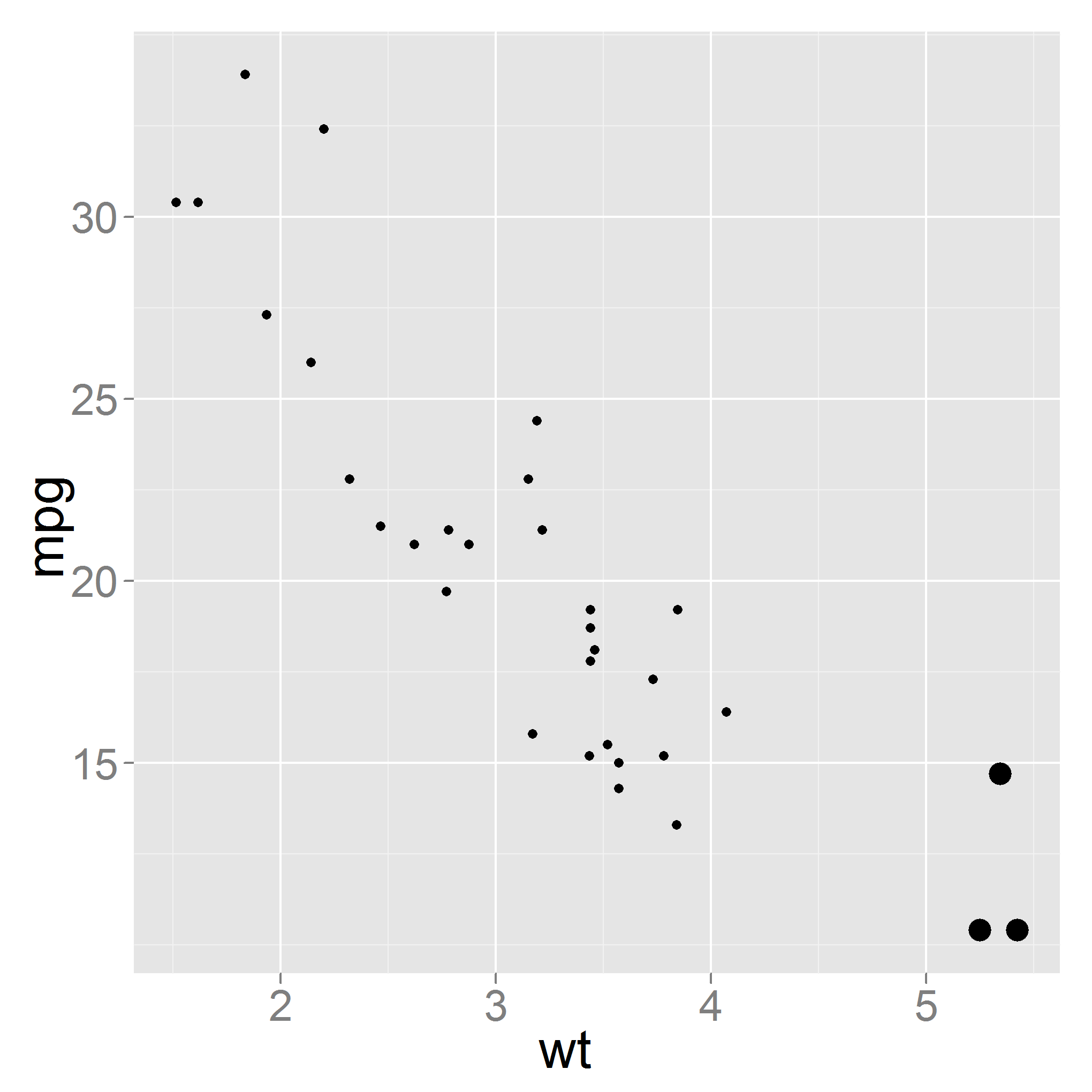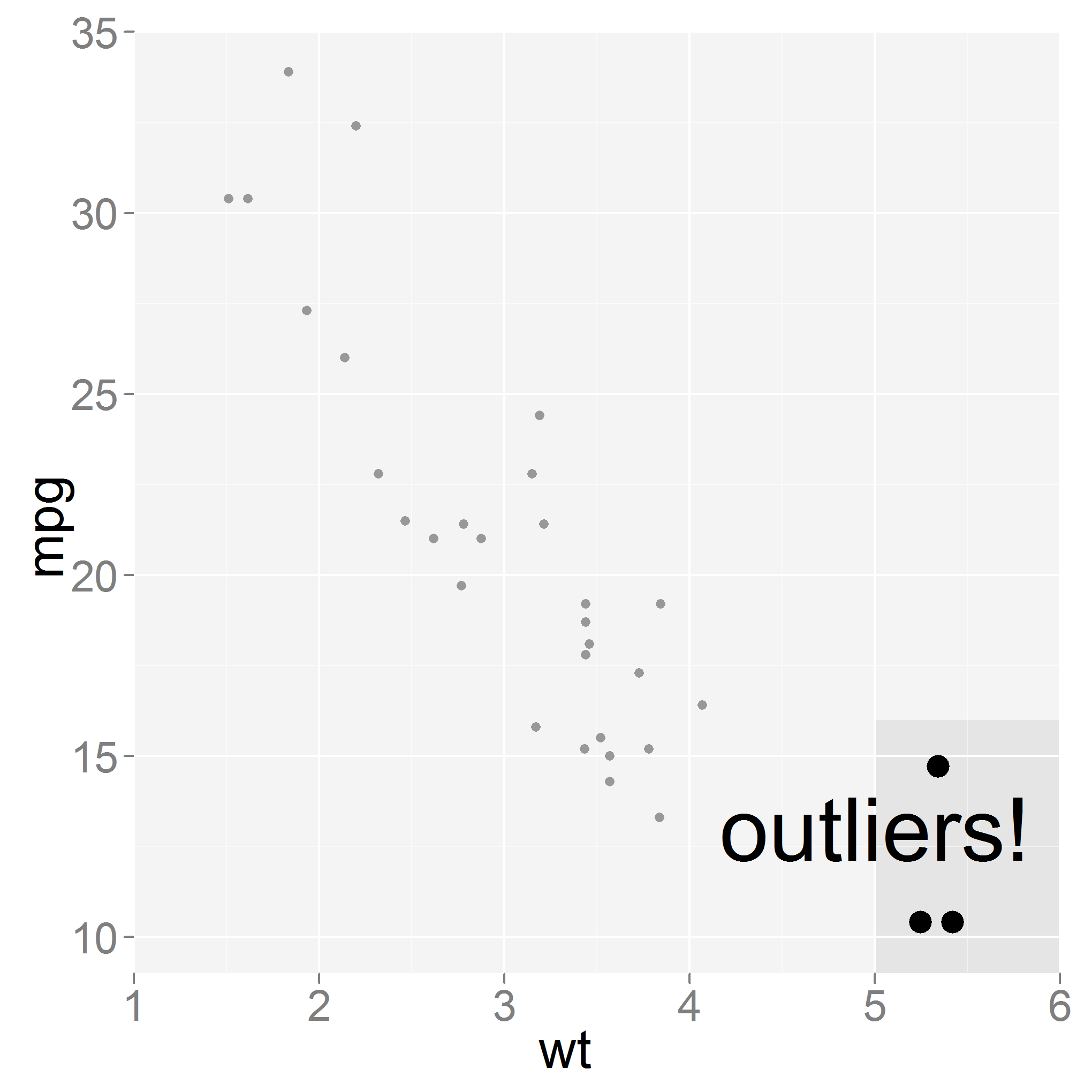Want to share your content on R-bloggers? click here if you have a blog, or here if you don't.

I’ve just finished reading slide:ology by Nancy Duarte. It contains lots of advice about how to convey meaning through aesthetics. The book has a general/business presentation focus, but it got me wondering about how to apply the ideas in a scientific context.  Since graphs from a big part of most scientific talks, and since that’s the bit I know best; that’s what I’m going to discuss here.

```p_basic <- ggplot(mtcars, aes(wt, mpg)) + geom_point()
```Something I’ve been burned with recently is overestimating the size of the projector screen. Although my graphs looked great when my face was next to my monitor, the axes were hard to read when projected. A good rule of thumb for text is your font size should be half the age of the eldest member of your audience or 30 points, whichever is bigger. For graphs axes we can perhaps get away with something a little smaller. Don’t forget that by the time you’ve printed your graph to file and played with it in your presentation software, the font size you’ve picked may bear no relation to it’s final value. That said, the point remains that you need to make the text bigger.

```old_theme <- theme_set(theme_grey(base_size = 24))
```Reading a graph can be quite an in depth process which can overwhelm you audience. Once you’ve shown them the graph in its basic form, you should emphasis interesting features, one at a time. The one at a time thing is important – if you want your audience to be concentrating, then you shouldn’t distract them with many things at once. Here I’ve increased the size of the outliers in the bottom right hand corner.

```mtcars <- within(mtcars, emph <- wt > 5)

p_emph <- ggplot(mtcars) +
geom_point(aes(wt, mpg, size = emph)) +
opts(legend.position = "none") +
scale_size_manual(values = c(2, 5))
```As well as emphasising values, a useful tool can be to de-emphasise other regions of the graph. Overlaying a translucent white rectangle on such regions does the trick nicely.

```x_lower <- floor(min(mtcars\$wt))
x_upper <- ceiling(max(mtcars\$wt))
y_lower <- floor(min(mtcars\$mpg))  -1
y_upper <- ceiling(max(mtcars\$mpg)) +1

p_deemph <- p_emph +
geom_rect(
aes(xmin = xmin, xmax = xmax, ymin = ymin, ymax = ymax),
data = data.frame(
xmin = c(x_lower, 5),
xmax = c(5, x_upper),
ymin = c(y_lower, 16),
ymax = rep.int(y_upper, 2)
),
fill = "white", alpha = 0.6
) +
scale_x_continuous(limits = c(x_lower, x_upper), expand = c(0, 0)) +
scale_y_continuous(limits = c(y_lower, y_upper), expand = c(0, 0))
```For more deemphsising more complicated regions, I find it easier to use dedicated image manipulation software. Paint.NET is my personal zero-cost favourite but any graphics software should suffice.

Finally, it can be useful to annotate your graph.

```p_annotated <- p_deemph +
geom_text(
aes(x = x, y = y, label = label),
data = data.frame(x = 5, y = 13, label = "outliers!"),
size = 14
)
```By now, if your audience hasn’t figured out which bit of the graph you want them to look at you’re in trouble. Let me know in the comments if you have any other ideas for how to draw graphs for presentations.

Tagged: dataviz, r